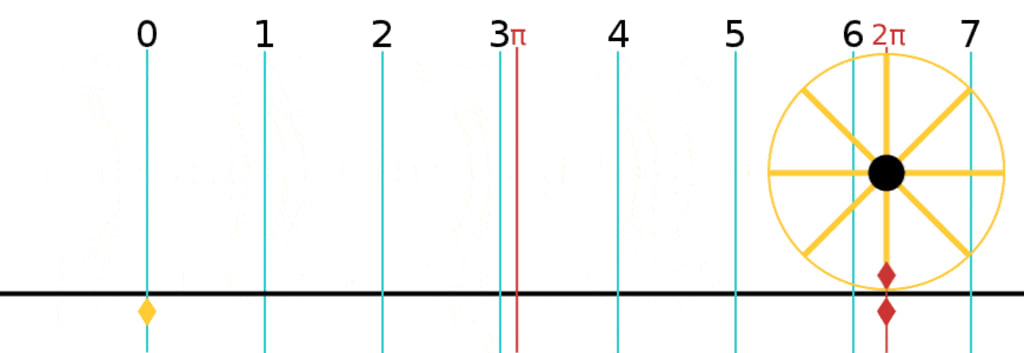# Pi is the Invention of Wheel in Mathematics

## 22/7 or 3.14

By Maria Rahman MalikPublished 2 months ago 3 min read
LikeWho invented the wheel is unknown to humans. Historically, the use of wheels or round objects began in prehistoric times when humans used them for transportation. These objects were typically made from stone or wood and were used to help move heavy loads across long distances. The earliest known example of a wheel comes from ancient Mesopotamia (around 3500 BC). These early wheels made from solid wood had been designed for carts or chariots.

The development of the wheel had revolutionized human productivity in terms of transportation and creating mechanical marvels.

Practically inventing and using the wheel may be easier as it was necessity to be invented by humans for ease of transportation against friction, therefore, it can be traced way back to prehistoric times. However, defining the wheel theoretically or explaining the circle mathematically was not that easy. Humans developed measuring methods for straight lines, squares, rectangles, or even triangles but measuring circle was complex. Mathematicians tried to measure "Circle" through "Square" the term known as "Squaring the Circle."

The winning idea was to find the distance a point on wheel covers on the ground for a given diameter in one revolution (known as the circumference of a circle). Of course, a large dia wheel will cover more, and a small dia wheel will cover less. To keep it simple, considering a wheel of dia of 1 m, the distance it will cover in one revolution is 3.14.... or 22/7 or Pi.

The calculation of Pi, or π, has revolutionized mathematics as the wheel has done to human life. Since the calculation of Pi, the circumference of a circle, its area, the volume of a sphere, cylinder, and many others, all were defined theoretically. However, finding the value of Pi was not that easy.

Ancient Babylonians of Mesepotomia (present day Iraq) were the first to define the the concept of Pi around 2000 BCE. They were the first to realize that the ratio of the circumference of a circle to its diameter was a constant, which they approximated to be around 3.125.

The ancient Egyptians also had a good understanding of the concept of Pi. They used it to design the pyramids, which required precise measurements to ensure their stability. They used a value of Pi that was close to 3.16.

The Greeks, however, were the first to explore the mathematical properties of Pi. Around 250 BCE, the mathematician Archimedes used a series of polygons to approximate the value of Pi. He calculated value of Pi between 3.1408 and 3.1429, which was a much more accurate estimate than before.

In the centuries that followed, mathematicians continued to refine their understanding of Pi. The Chinese mathematician Zu Chongzhi, who lived in the 5th century CE, calculated Pi to six decimal places, a remarkable achievement for that time.

Over the years, the quest for a more precise value of Pi continued. John Wallis, an English Mathematician developed a formula for calculating Pi in 17th Century. This formula allowed mathematicians to calculate Pi to any desired degree of accuracy.

Pi has revolutionized science and technology as Wheel has revolutionized human history. "Creation of Pi" is explained in this article as "Invention of Wheel in Mathematics." For example, Pi is used to define the size of planets and stars in astronomy, to calculate the frequency and period of waves in electromagnetism, to define the probability of events, and design circular objects in geometry are few use of Pi in today's world.

Today, the value of Pi is known to over 31 trillion decimal places, thanks to the use of powerful computers and sophisticated algorithms. Despite its long history and many refinements, the value of Pi remains an object of fascination and wonder for mathematicians and non-mathematicians alike.

Imagine a life without a wheel or circular objects in your life. We will be struggling with friction with straight-shaped objects. Similarly, Mathematics without Pi will be struggling to achieve the marvels it has achieved today. It is safe to say that "Pi is the Invention of the Wheel in Mathematics."

fact or fiction
Like

### Maria Rahman Malik

How does it work?

There are no comments for this story

Be the first to respond and start the conversation.